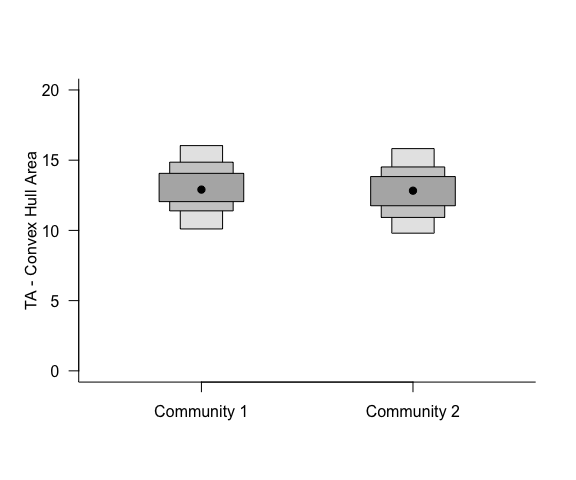# Introduction to SIBER

## SIBER introduction and guide

This user manual introduces the basic functionality of this standalone version of SIBER, which was previously part of the siar package. SIBER contains two types of analysis, although both are founded on the same principle of fitting ellipses to groups of data. The questions are very different though and it is important that you satisfy yourself with which one you want for the questions you have of your own data. There are additional learning resources available at SIAR-examples-and-queries. N.B. these examples currently still use the SIBER functions embedded within the SIAR package, which I will update shortly, but the concepts are exactly the same.

## Setting up our R session for this demonstration

In this example, we are going to work with a bundled example dataset that I created previously using the function generateSiberData(). This dataset is loaded using data("demo.siber.data") but it is also provided as a raw *.csv file which is more usually the format you would work with when organising your own dataset for analysis using SIBER. We then use createSiberObject() to convert this raw data form into an object that contains information summary statistics that are useful for plotting, and a z-score transformed version of the data which is used in the model fitting process before being back-transformed using the summary statistic information.

# remove previously loaded items from the current environment and remove previous graphics.
rm(list=ls())
graphics.off()

# Here, I set the seed each time so that the results are comparable.
# This is useful as it means that anyone that runs your code, *should*
# get the same results as you, although random number generators change
# from time to time.
set.seed(1)

library(SIBER)

# load in the included demonstration dataset
data("demo.siber.data")
#
# create the siber object
siber.example <- createSiberObject(demo.siber.data)

# Or if working with your own data read in from a *.csv file, you would use
# This *.csv file is included with this package. To find its location
# type
# fname <- system.file("extdata", "demo.siber.data.csv", package = "SIBER")
# in your command window. You could load it directly by using the
# returned path, or perhaps better, you could navigate to this folder
# and copy this file to a folder of your own choice, and create a
# script from this vingette to analyse it. This *.csv file provides
# a template for how your own files should be formatted.

# siber.example <- createSiberObject(mydata)

## Plotting the raw data

With the siber object created, we can now use various functions to create isotope biplots of the data, and also calculate some summary statistics on each group and/or community in the dataset.

Community 1 comprises 3 groups and drawn as black, red and green circles community 2 comprises 3 groups and drawn as black, red and green triangles

Various plotting options are collated into lists and then passed to the high-level SIBER plotting function plotSiberObject which is a wrapper function for easy plotting. We will access the more specific plotting functions directly a little later on to create more customised graphics.

ax.pad determines the padding applied around the extremes of the data.

iso.order is a vector of length 2 specifying which isotope should be plotted on the x and y axes.N.B. there is currently a problem with the addition of the group ellipses using if you deviate from the default of iso.order = c(1,2). This argument will be deprecated in a future release, and plotting order will be achieved at point of data-entry. I recommend you set up your original data with the chemical element you want plotted on the x-axis being the first column, and the y-axis in the second.

Convex hulls are drawn between the centres of each group within a community with hulls = T.

Ellipses are drawn for each group independently with ellipses = T. These ellipses can be made to be maximum likelihood standard ellipses by setting p = NULL, or can be made to be prediction ellipses that contain approximately p proportion of data. For example, p = 0.95 will draw an ellipse that encompasses approximately 95% of the data. The parameter n determines how many points are used to make each ellipse and hence how smooth the curves are.

Convex hulls are draw around each group independently with group.hulls = T.

# Create lists of plotting arguments to be passed onwards to each
# of the three plotting functions.
community.hulls.args <- list(col = 1, lty = 1, lwd = 1)
group.ellipses.args  <- list(n = 100, p.interval = 0.95, lty = 1, lwd = 2)
group.hull.args      <- list(lty = 2, col = "grey20")

par(mfrow=c(1,1))
plotSiberObject(siber.example,
hulls = F, community.hulls.args,
ellipses = T, group.ellipses.args,
group.hulls = T, group.hull.args,
bty = "L",
iso.order = c(1,2),
xlab = expression({delta}^13*C~'\u2030'),
ylab = expression({delta}^15*N~'\u2030')
)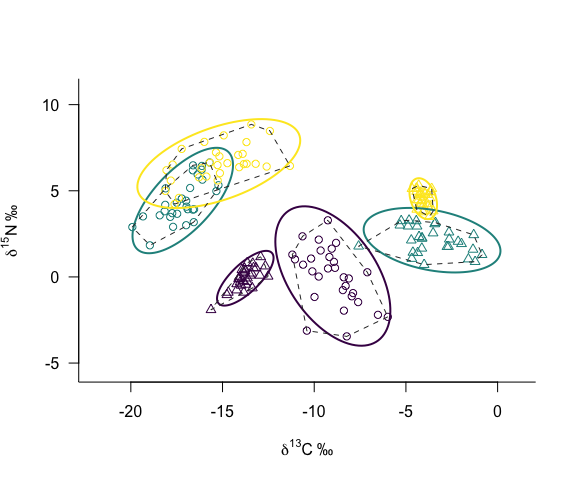## Summary statistics and custom graphic additions

Although the intention of SIBER is to use Bayesian methods to allow us to make statistical comparisons of what are otherwise typically point estimates of dispersion within and among communities and groups, the basic summary statistics are informative and useful for checking that our Bayesian analysis is working as intended.

One feature of the Standard Ellipse is that it contains approximately 40% of the data. SIBER now includes code to scale this ellipse so that it contains approximately any % of the data you wish. Additionally, the ellipse can be scaled so that it represents a % confidence ellipse of the bivariate means (rather than of the data). We create the bi-plot again here and this time add the additional ellipses overlayed on the basic plot that this time omits group hulls and group standard ellipses.


par(mfrow=c(1,1))

community.hulls.args <- list(col = 1, lty = 1, lwd = 1)
group.ellipses.args  <- list(n = 100, p.interval = 0.95, lty = 1, lwd = 2)
group.hull.args      <- list(lty = 2, col = "grey20")

# this time we will make the points a bit smaller by
# cex = 0.5
plotSiberObject(siber.example,
hulls = F, community.hulls.args,
ellipses = F, group.ellipses.args,
group.hulls = F, group.hull.args,
bty = "L",
iso.order = c(1,2),
xlab=expression({delta}^13*C~'\u2030'),
ylab=expression({delta}^15*N~'\u2030'),
cex = 0.5
)

# Calculate summary statistics for each group: TA, SEA and SEAc
group.ML <- groupMetricsML(siber.example)
print(group.ML)
#>            1.1       1.2       1.3       2.1       2.2       2.3
#> TA   21.924922 10.917715 17.945127 3.0714363 11.476354 1.4818061
#> SEA   5.783417  3.254484  5.131601 0.8623300  3.458824 0.4430053
#> SEAc  5.989967  3.370715  5.314872 0.8931275  3.582354 0.4588269

# You can add more ellipses by directly calling plot.group.ellipses()
plotGroupEllipses(siber.example, n = 100, p.interval = 0.95,
lty = 1, lwd = 2)

# or you can add the XX% confidence interval around the bivariate means
# by specifying ci.mean = T along with whatever p.interval you want.
plotGroupEllipses(siber.example, n = 100, p.interval = 0.95, ci.mean = T,
lty = 1, lwd = 2)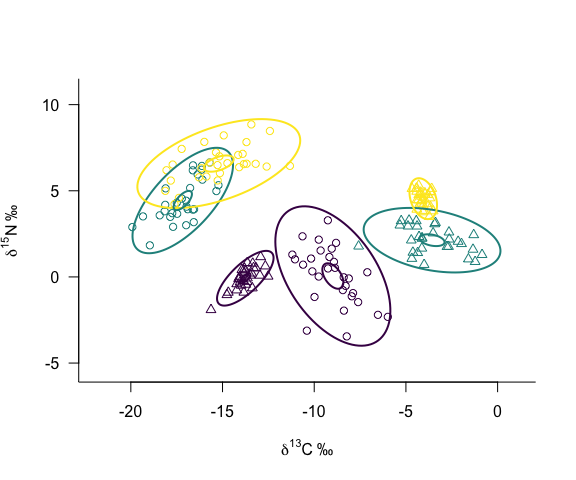Alternatively, we may wish to focus on comparing the two communities represented in these plots by the open circles and the open triangles. To illustrate these groupings, we might draw the convex hull between the means of each of the three groups comprising each community. Additionally, I have highlighted the location of each group by adding the 95% confidence interval of their bivariate mean.

# A second plot provides information more suitable to comparing
# the two communities based on the community-level Layman metrics

# this time we will make the points a bit smaller by
# cex = 0.5
plotSiberObject(siber.example,
hulls = T, community.hulls.args,
ellipses = F, group.ellipses.args,
group.hulls = F, group.hull.args,
bty = "L",
iso.order = c(1,2),
xlab=expression({delta}^13*C~'\u2030'),
ylab=expression({delta}^15*N~'\u2030'),
cex = 0.5
)

# or you can add the XX% confidence interval around the bivariate means
# by specifying ci.mean = T along with whatever p.interval you want.
plotGroupEllipses(siber.example, n = 100, p.interval = 0.95,
ci.mean = T, lty = 1, lwd = 2)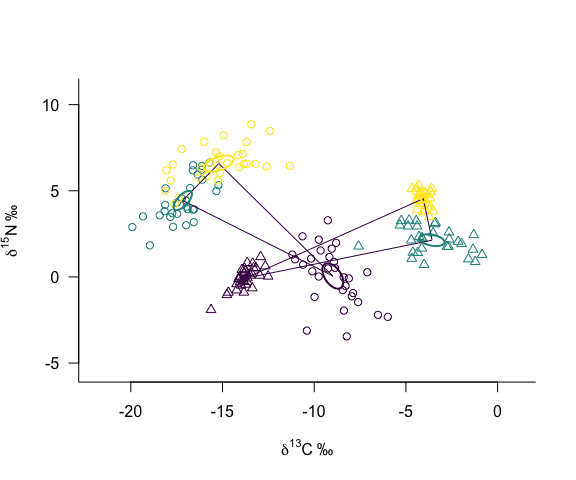# Calculate the various Layman metrics on each of the communities.
community.ML <- communityMetricsML(siber.example)
print(community.ML)
#>                  1         2
#> dY_range  6.526633  4.617564
#> dX_range  8.184069 10.184171
#> TA       13.079701 12.799232
#> CD        4.235518  4.809830
#> MNND      4.944052  5.111483
#> SDNND     3.526088  4.598012

# Fitting the Bayesian models to the data

Whether your intended analysis is to compare isotopic niche width among groups, or among communities, the initial step is to fit Bayesian multivariate normal distributions to each group in the dataset. The decision as to whether you then want to compare the area of the ellipses among groups, or any / all of the 6 Layman metrics comes later.

These multivariate normal distributions are fitted using the jags software run via the package rjags. This method relies on an iterated Gibbs Sampling technique and some information on the length, number and iterations of sampling chains is required. Additionally, the prior distributions for the parameters need to be specified. In SIBER, these are bundled into two list objects: parms which holds the parameters defining how the sampling algorithm is to run; and priors which holds information on the prior distributions of the parameters to be estimated. Typically, the priors are left vague and you should use these same values in your own analysis. Since the data are z-scored internally before the models are fitted to the data, the expected means are inherently close to zero, and the marginal variances close to one. This greatly aids the jags fitting process.

After calling siberMVN() you will see output in the command window indicating that the jags models are being fitted, one block of output for each group in your dataset. A subset of these blocks are shown below.


# options for running jags
parms <- list()
parms$n.iter <- 2 * 10^4 # number of iterations to run the model for parms$n.burnin <- 1 * 10^3 # discard the first set of values
parms$n.thin <- 10 # thin the posterior by this many parms$n.chains <- 2        # run this many chains

# define the priors
priors <- list()
priors$R <- 1 * diag(2) priors$k <- 2
priors$tau.mu <- 1.0E-3 # fit the ellipses which uses an Inverse Wishart prior # on the covariance matrix Sigma, and a vague normal prior on the # means. Fitting is via the JAGS method. ellipses.posterior <- siberMVN(siber.example, parms, priors) #> Compiling model graph #> Resolving undeclared variables #> Allocating nodes #> Graph information: #> Observed stochastic nodes: 30 #> Unobserved stochastic nodes: 3 #> Total graph size: 45 #> #> Initializing model #> #> Compiling model graph #> Resolving undeclared variables #> Allocating nodes #> Graph information: #> Observed stochastic nodes: 30 #> Unobserved stochastic nodes: 3 #> Total graph size: 45 #> #> Initializing model #> #> Compiling model graph #> Resolving undeclared variables #> Allocating nodes #> Graph information: #> Observed stochastic nodes: 30 #> Unobserved stochastic nodes: 3 #> Total graph size: 45 #> #> Initializing model #> #> Compiling model graph #> Resolving undeclared variables #> Allocating nodes #> Graph information: #> Observed stochastic nodes: 30 #> Unobserved stochastic nodes: 3 #> Total graph size: 45 #> #> Initializing model #> #> Compiling model graph #> Resolving undeclared variables #> Allocating nodes #> Graph information: #> Observed stochastic nodes: 30 #> Unobserved stochastic nodes: 3 #> Total graph size: 45 #> #> Initializing model #> #> Compiling model graph #> Resolving undeclared variables #> Allocating nodes #> Graph information: #> Observed stochastic nodes: 30 #> Unobserved stochastic nodes: 3 #> Total graph size: 45 #> #> Initializing model ## Comparing among groups using the Standard Ellipse Area When comparing individual groups with each other, be it within a single community, or groups among communities, the Standard Ellipse Area (SEA) is the recommended method. Since the multivariate normal distributions have already been fitted to each group, it only remains to calculate the SEA on the posterior distribution of covariance matrix for each group, thereby yielding the Bayesian SEA or SEA-B. We can also use the summary statistics we calculated earlier to add the maximum likelihood estimates of SEA-c to the Bayesian estimates. Plotting is via the function siberDensityPlot() which is essentially the same as siardensityplot() from the older version of SIAR. Credible intervals can be extracted by calling the function hdr from the hdrcde package.  # The posterior estimates of the ellipses for each group can be used to # calculate the SEA.B for each group. SEA.B <- siberEllipses(ellipses.posterior) siberDensityPlot(SEA.B, xticklabels = colnames(group.ML), xlab = c("Community | Group"), ylab = expression("Standard Ellipse Area " ('\u2030' ^2) ), bty = "L", las = 1, main = "SIBER ellipses on each group" ) # Add red x's for the ML estimated SEA-c points(1:ncol(SEA.B), group.ML[3,], col="red", pch = "x", lwd = 2)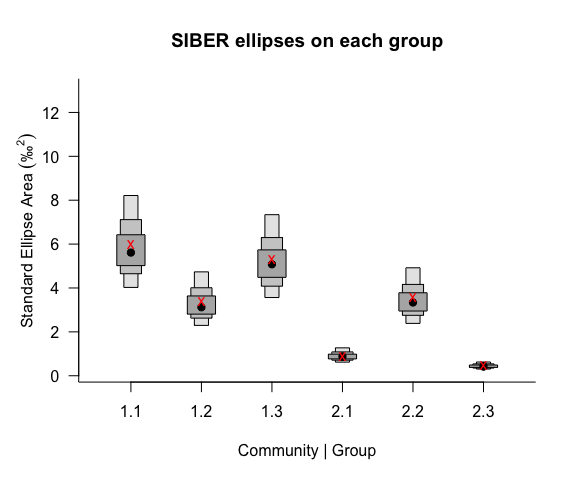# Calculate some credible intervals cr.p <- c(0.95, 0.99) # vector of quantiles # call to hdrcde:hdr using lapply() SEA.B.credibles <- lapply( as.data.frame(SEA.B), function(x,...){tmp<-hdrcde::hdr(x)$hdr},
prob = cr.p)

# do similar to get the modes, taking care to pick up multimodal posterior
# distributions if present
SEA.B.modes <- lapply(
as.data.frame(SEA.B),
function(x,...){tmp<-hdrcde::hdr(x)$mode}, prob = cr.p, all.modes=T) ## Comparison of entire communities using the Layman metrics Entire communities can be compared by recognising that convex hulls (or any other metric) based on the centroids of each group are subject to uncertainty in the location of the centroids. We can exploit this and calculate the distribution of convex hull areas (and the other 5 metrics) based on the posterior distribution of the means of each group’s x and y data. The first thing to do is to extract the posterior means from the mcmc.list object that jags creates to make plotting and analysis easier. Thereafter, we can plot their distribution using highest density region boxplots to visualise the credible intervals that describe it.  # extract the posterior means mu.post <- extractPosteriorMeans(siber.example, ellipses.posterior) # calculate the corresponding distribution of layman metrics layman.B <- bayesianLayman(mu.post) # -------------------------------------- # Visualise the first community # -------------------------------------- siberDensityPlot(layman.B[], xticklabels = colnames(layman.B[]), bty="L", ylim = c(0,20)) # add the ML estimates (if you want). Extract the correct means # from the appropriate array held within the overall array of means. comm1.layman.ml <- laymanMetrics(siber.example$ML.mu[][1,1,],
siber.example$ML.mu[][1,2,] ) points(1:6, comm1.layman.ml$metrics, col = "red", pch = "x", lwd = 2)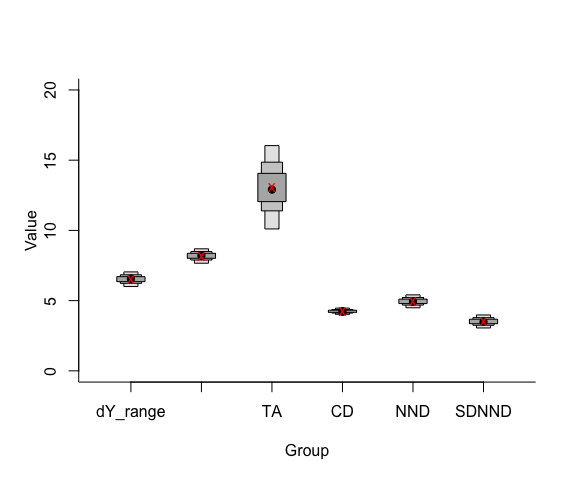# --------------------------------------
# Visualise the second community
# --------------------------------------
siberDensityPlot(layman.B[], xticklabels = colnames(layman.B[]),
bty="L", ylim = c(0,20))

# add the ML estimates. (if you want) Extract the correct means
# from the appropriate array held within the overall array of means.
comm2.layman.ml <- laymanMetrics(siber.example$ML.mu[][1,1,], siber.example$ML.mu[][1,2,]
)
points(1:6, comm2.layman.ml\$metrics, col = "red", pch = "x", lwd = 2)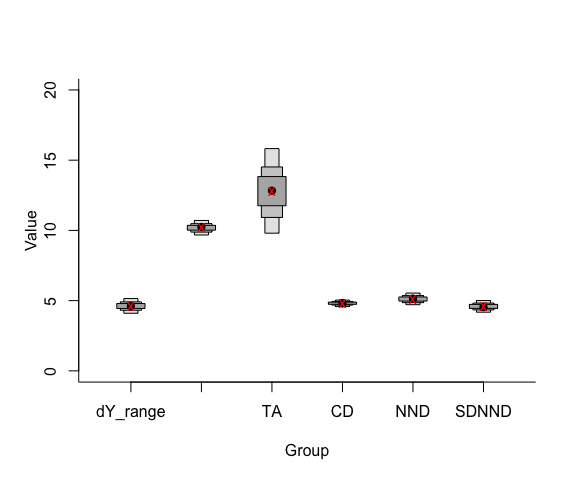# --------------------------------------
# Alternatively, pull out TA from both and aggregate them into a
# single matrix using cbind() and plot them together on one graph.
# --------------------------------------

# go back to a 1x1 panel plot
par(mfrow=c(1,1))

siberDensityPlot(cbind(layman.B[][,"TA"], layman.B[][,"TA"]),
xticklabels = c("Community 1", "Community 2"),
bty="L", ylim = c(0,20),
las = 1,
ylab = "TA - Convex Hull Area",
xlab = "")